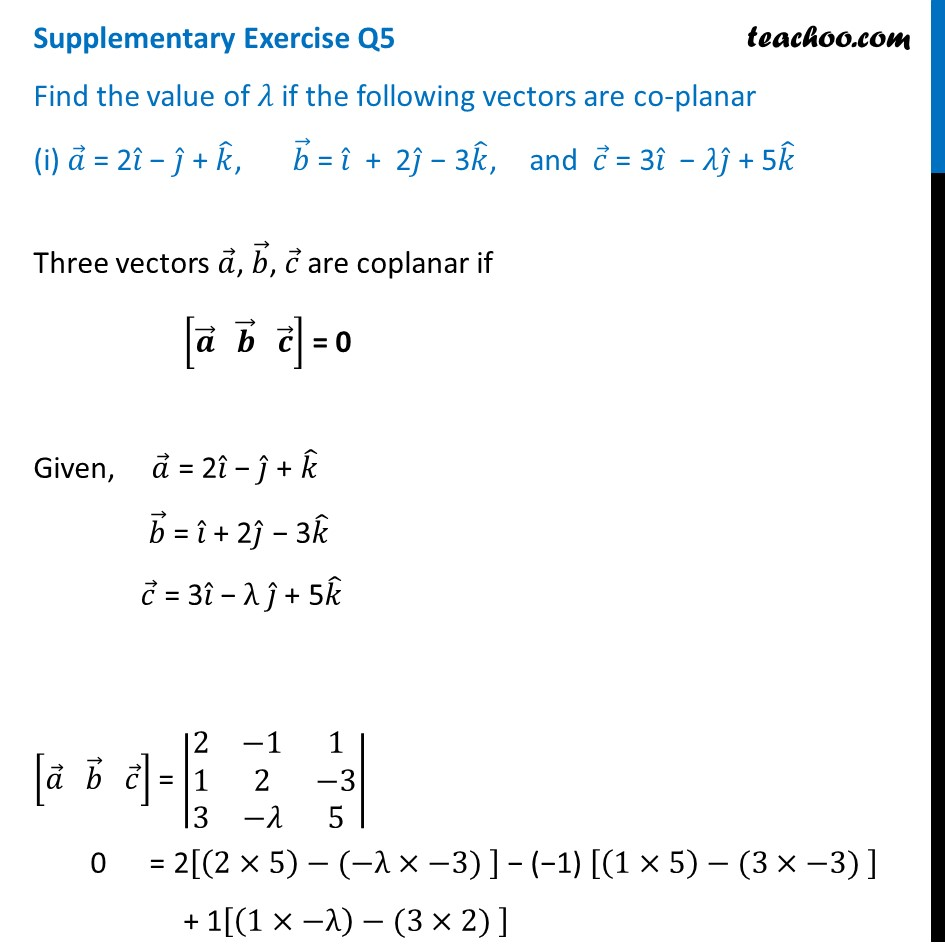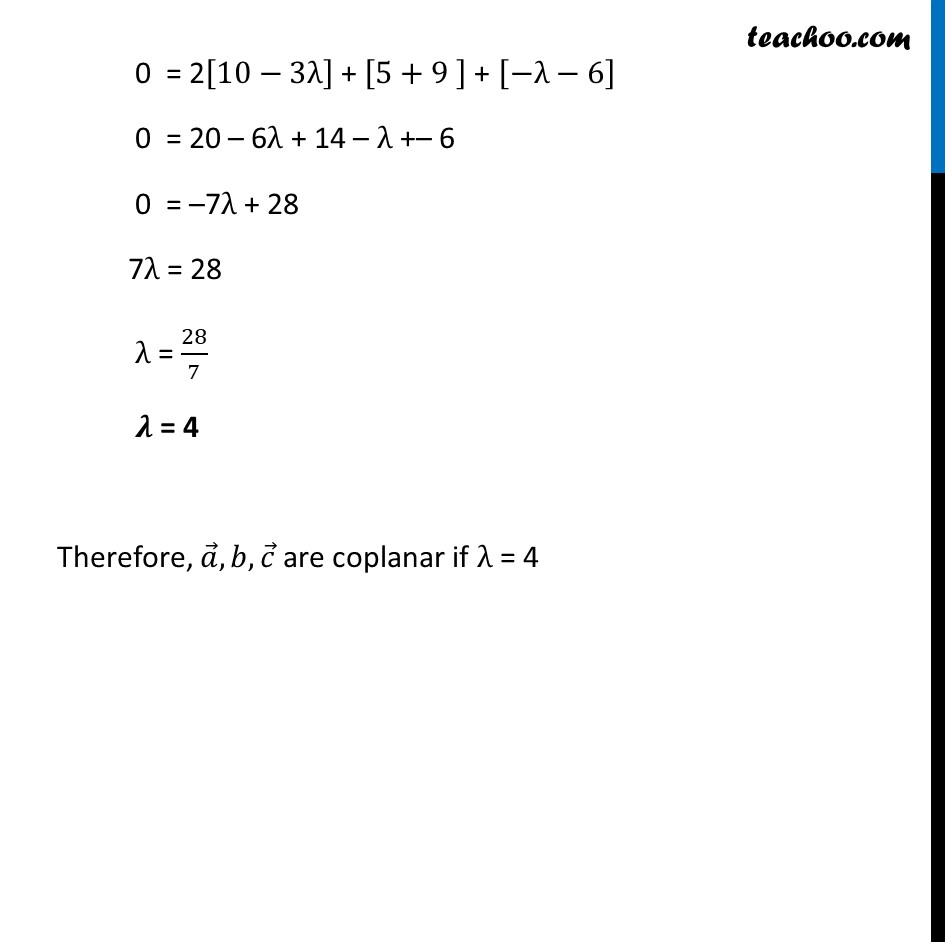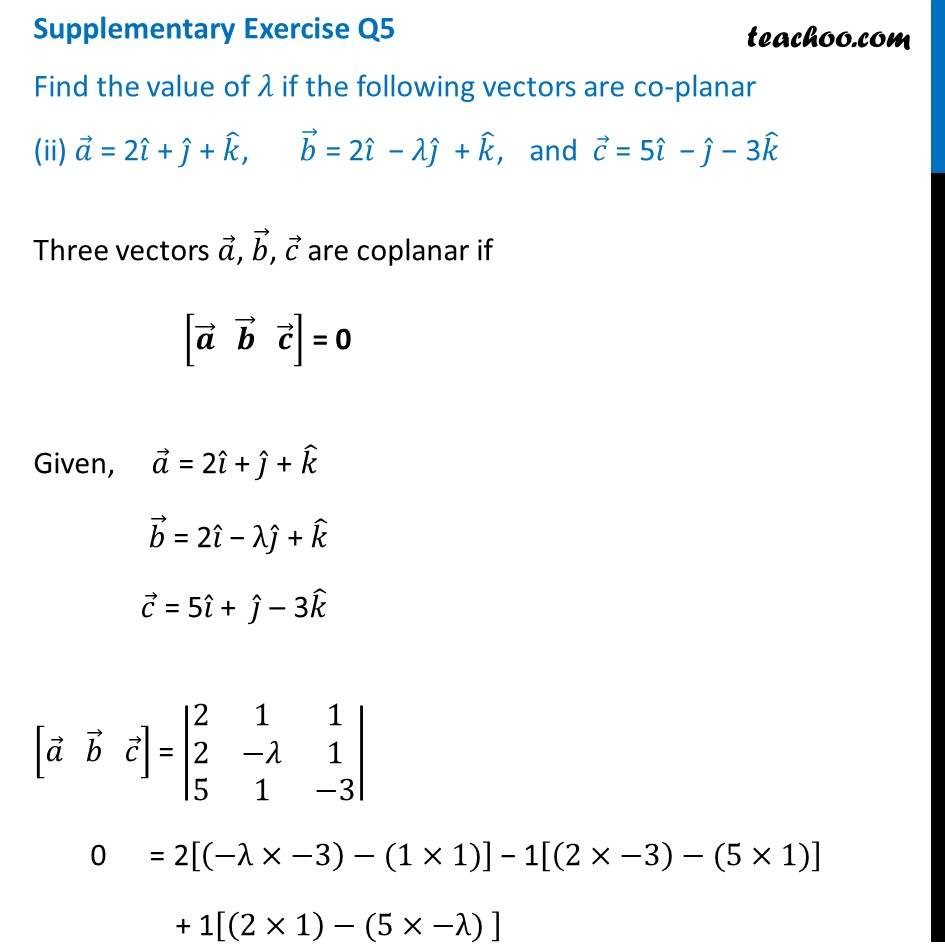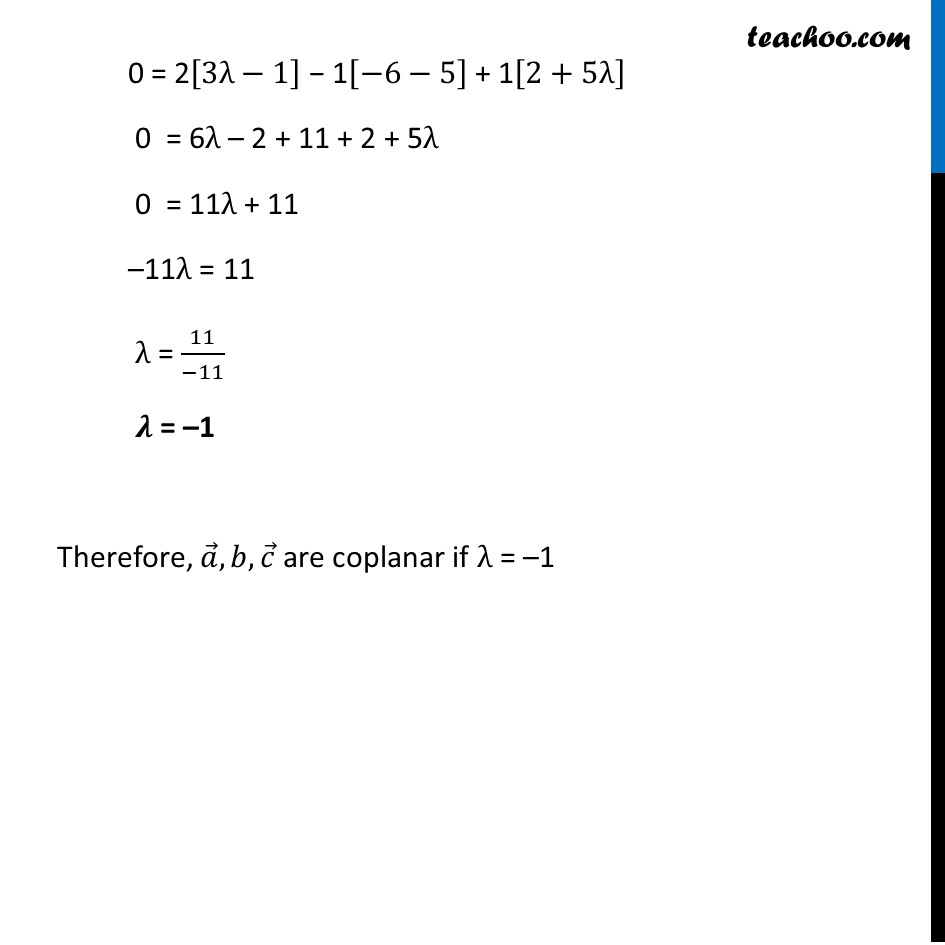Supplementary examples and questions from CBSE

Chapter 10 Class 12 Vector Algebra
Serial order wiseLearn in your speed, with individual attention - Teachoo Maths 1-on-1 Class

### Transcript

Supplementary Exercise Q5 Find the value of 𝜆 if the following vectors are co-planar (i) 𝑎 ⃗ = 2𝑖 ̂ − 𝑗 ̂ + 𝑘 ̂, 𝑏 ⃗ = 𝑖 ̂ + 2𝑗 ̂ − 3𝑘 ̂, and 𝑐 ⃗ = 3𝑖 ̂ − 𝜆𝑗 ̂ + 5𝑘 ̂ Three vectors 𝑎 ⃗, 𝑏 ⃗, 𝑐 ⃗ are coplanar if [𝒂 ⃗" " 𝒃 ⃗" " 𝒄 ⃗ ] = 0 Given, 𝑎 ⃗ = 2𝑖 ̂ − 𝑗 ̂ + 𝑘 ̂ 𝑏 ⃗ = 𝑖 ̂ + 2𝑗 ̂ − 3𝑘 ̂ 𝑐 ⃗ = 3𝑖 ̂ − λ 𝑗 ̂ + 5𝑘 ̂ [𝑎 ⃗" " 𝑏 ⃗" " 𝑐 ⃗ ] = |■8(2&−1&1@1&2&−3@3&−𝜆&5)| 0 = 2[(2×5)−(−λ×−3) ] − (−1) [(1×5)−(3×−3) ] + 1[(1×−λ)−(3×2) ] 0 = 2[10−3λ] + [5+9 ] + [−λ−6] 0 = 20 – 6λ + 14 – λ +– 6 0 = –7λ + 28 7λ = 28 λ = 28/7 𝝀 = 4 Therefore, 𝑎 ⃗,𝑏,𝑐 ⃗ are coplanar if λ = 4 Supplementary Exercise Q5 Find the value of 𝜆 if the following vectors are co-planar (ii) 𝑎 ⃗ = 2𝑖 ̂ + 𝑗 ̂ + 𝑘 ̂, 𝑏 ⃗ = 2𝑖 ̂ − 𝜆𝑗 ̂ + 𝑘 ̂, and 𝑐 ⃗ = 5𝑖 ̂ − 𝑗 ̂ − 3𝑘 ̂ Three vectors 𝑎 ⃗, 𝑏 ⃗, 𝑐 ⃗ are coplanar if [𝒂 ⃗" " 𝒃 ⃗" " 𝒄 ⃗ ] = 0 Given, 𝑎 ⃗ = 2𝑖 ̂ + 𝑗 ̂ + 𝑘 ̂ 𝑏 ⃗ = 2𝑖 ̂ − λ𝑗 ̂ + 𝑘 ̂ 𝑐 ⃗ = 5𝑖 ̂ + 𝑗 ̂ – 3𝑘 ̂ [𝑎 ⃗" " 𝑏 ⃗" " 𝑐 ⃗ ] = |■8(2&1&1@2&−𝜆&1@5&1&−3)| 0 = 2[(−λ×−3)−(1×1)] − 1[(2×−3)−(5×1)] + 1[(2×1)−(5×−λ) ] 0 = 2[3λ−1] − 1[−6−5] + 1[2+5λ] 0 = 6λ – 2 + 11 + 2 + 5λ 0 = 11λ + 11 –11λ = 11 λ = 11/(−11) 𝝀 = –1 Therefore, 𝑎 ⃗,𝑏,𝑐 ⃗ are coplanar if λ = –1